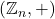# Some applications of idempotent elements in MV algebras

### Full PDFcarpathian_2023_39_1_161_174

In this paper we provide some properties and applications of MV-algebras. We prove that a Fibonacci stationary sequence in an MV-algebra gives us an idempotent element. Moreover, taking into
account of the representation of a finite MV-algebra, by using Boolean elements of this algebra, we prove that a Fibonacci sequence in an MV-algebra is always stationary. This result is interesting comparing with
the behavior of such a sequence on the group, where the Fibonacci sequences are periodic, with the period given by the Pisano period. We also give some examples of finite MV-algebras and the number of their idempotent elements. As an application in Coding Theory, to a Boolean algebra it is attached a binary block code and it is proved that, under some conditions, the converse is also true.